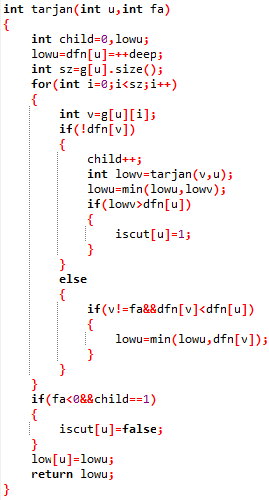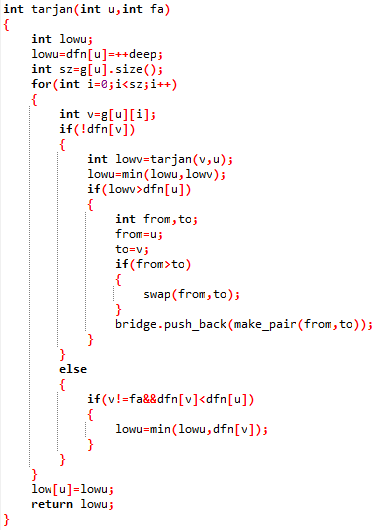# tarjan求强连通分量+缩点+割点/割桥（点双/边双）以及一些证明

“tarjan陪伴强联通分量

1、什么是强连通分量？

“有向图强连通分量：在有向图G中，如果两个顶点vi,vj间（vi>vj）有一条从vi到vj的有向路径，同时还有一条从vj到vi的有向路径，则称两个顶点强连通(strongly connected)。如果有向图G的每两个顶点都强连通，称G是一个强连通图。有向图的极大强连通子图，称为强连通分量(strongly connected components)。”2、强连通分量怎么找？(1)、dfn[ ]，表示这个点在dfs时是第几个被搜到的。

(2)、low[ ]，表示这个点以及其子孙节点连的所有点中dfn最小的值

(3)、stack[ ]，表示当前所有可能能构成是强连通分量的点。

(4)、vis[ ]，表示一个点是否在stack[ ]数组中。

(1)、首先初始化dfn[u]=low[u]=第几个被dfs到

dfn可以理解，但为什么low也要这么做呢？

因为low的定义如上，也就是说如果没有子孙与u的祖先相连的话，dfn[u]一定是它和它的所有子孙中dfn最小的（因为它的所有子孙一定比他后搜到）

(2)、将u存入stack[ ]中，并将vis[u]设为true

stack[ ]有什么用？

(3)、遍历u的每一个能到的点，如果这个点dfn[ ]为0，即仍未访问过，那么就对点v进行dfs，然后low[u]=min{low[u],low[v]}

low[ ]有什么用？

(4)、假设我们已经dfs完了u的所有的子树那么之后无论我们再怎么dfs，u点的low值已经不会再变了。

dfn表示u点被dfs到的时间，low表示u和u所有的子树所能到达的点中dfn最小的。

tarjan到此结束[USACO06JAN]牛的舞会The Cow Prom

给你n个点，m条边，求图中所有大小大于1的强连通分量的个数

5 4
2 4
3 5
1 2
4 1

1

#include<queue>
#include<cstdio>
#include<vector>
#include<cstring>
#include<iostream>
#include<algorithm>
using namespace std;
#define inf 0x3f3f3f3f

vector<int> g;
int color,dfn,low,stack,vis,cnt;
int deep,top,n,m,sum,ans;

void tarjan(int u)
{
dfn[u]=++deep;
low[u]=deep;
vis[u]=1;
stack[++top]=u;
int sz=g[u].size();
for(int i=0;i<sz;i++)
{
int v=g[u][i];
if(!dfn[v])
{
tarjan(v);
low[u]=min(low[u],low[v]);
}
else
{
if(vis[v])
{
low[u]=min(low[u],low[v]);
}
}
}
if(dfn[u]==low[u])
{
color[u]=++sum;
vis[u]=0;
while(stack[top]!=u)
{
color[stack[top]]=sum;
vis[stack[top--]]=0;
}
top--;
}
}

int main()
{
scanf("%d%d",&n,&m);
for(int i=1;i<=m;i++)
{
int from,to;
scanf("%d%d",&from,&to);
g[from].push_back(to);
}
for(int i=1;i<=n;i++)
{
if(!dfn[i])
{
tarjan(i);
}
}
for(int i=1;i<=n;i++)
{
cnt[color[i]]++;
}
for(int i=1;i<=sum;i++)
{
if(cnt[i]>1)
{
ans++;
}
}
printf("%d\n",ans);
}poj2186 Popular Cows

Sample Input
3 3
1 2
2 1
2 3
Sample Output
1

#include<cmath>
#include<cstdio>
#include<vector>
#include<cstring>
#include<iostream>
#include<algorithm>
using namespace std;

int dfn,low,vis,stack,color,du,cnt;
int n,m,top,sum,deep,tmp,ans;
vector<int> g;

void tarjan(int u)
{
dfn[u]=low[u]=++deep;
vis[u]=1;
stack[++top]=u;
int sz=g[u].size();
for(int i=0; i<sz; i++)
{
int v=g[u][i];
if(!dfn[v])
{
tarjan(v);
low[u]=min(low[u],low[v]);
}
else
{
if(vis[v])
{
low[u]=min(low[u],low[v]);
}
}
}
if(dfn[u]==low[u])
{
color[u]=++sum;
vis[u]=0;
while(stack[top]!=u)
{
color[stack[top]]=sum;
vis[stack[top--]]=0;
}
top--;
}
}

int main()
{
while(scanf("%d%d",&n,&m)!=EOF)
{
memset(vis,0,sizeof(du));
memset(vis,0,sizeof(low));
memset(dfn,0,sizeof(dfn));
memset(vis,0,sizeof(vis));
memset(vis,0,sizeof(cnt));
memset(vis,0,sizeof(color));
memset(vis,0,sizeof(stack));
for(int i=1; i<=n; i++)
{
g[i].clear();
}
for(int i=1; i<=m; i++)
{
int from,to;
scanf("%d%d",&from,&to);
g[from].push_back(to);
}
for(int i=1; i<=n; i++)
{
if(!dfn[i])
{
tarjan(i);
}
}
for(int i=1; i<=n; i++)
{
int sz=g[i].size();
for(int j=0; j<sz; j++)
{
int v=g[i][j];
if(color[v]!=color[i])
{
du[color[i]]++;
}
}
cnt[color[i]]++;
}
for(int i=1; i<=sum; i++)
{
if(du[i]==0)
{
tmp++;
ans=cnt[i];
}
}
if(tmp==0)
{
printf("0\n");

}
else
{
if(tmp>1)
{
printf("0\n");
}
else
{
printf("%d\n",ans);
}
}
}
}

1、什么是割点、桥2、割点和桥怎么求？#include<cstdio>
#include<vector>
#include<cstring>
#include<iostream>
#include<algorithm>
#define hi printf("hi!");
using namespace std;

vector<int> g;
int dfn,low,iscut,son;
int deep,root,n,m,ans;

int tarjan(int u,int fa)
{
int child=0,lowu;
lowu=dfn[u]=++deep;
int sz=g[u].size();
for(int i=0;i<sz;i++)
{
int v=g[u][i];
if(!dfn[v])
{
child++;
int lowv=tarjan(v,u);
lowu=min(lowu,lowv);
if(lowv>dfn[u])
{
iscut[u]=1;
}
}
else
{
if(v!=fa&&dfn[v]<dfn[u])
{
lowu=min(lowu,dfn[v]);
}
}
}
if(fa<0&&child==1)
{
iscut[u]=false;
}
low[u]=lowu;
return lowu;
}

int main()
{
scanf("%d%d",&n,&m);
for(int i=1;i<=m;i++)
{
int from,to;
scanf("%d%d",&from,&to);
g[from].push_back(to);
g[to].push_back(from);
}
for(int i=1;i<=n;i++)
{
if(!dfn[i])
{
root=i;
tarjan(i,-1);
}
}
for(int i=1;i<=n;i++)
{
if(iscut[i])
{
ans++;
}
}
printf("%d\n",ans);
for(int i=1;i<=n;i++)
{
if(iscut[i])
{
printf("%d ",i);
}
}
}#include<cstdio>
#include<vector>
#include<cstring>
#include<iostream>
#include<algorithm>
#define hi printf("hi!");
using namespace std;

vector<pair<int,int> >bridge;
vector<int> g;
int dfn,low;
int deep,root,n,m,ans;

int tarjan(int u,int fa)
{
int lowu;
lowu=dfn[u]=++deep;
int sz=g[u].size();
for(int i=0;i<sz;i++)
{
int v=g[u][i];
if(!dfn[v])
{
int lowv=tarjan(v,u);
lowu=min(lowu,lowv);
if(lowv>dfn[u])
{
int from,to;
from=u;
to=v;
if(from>to)
{
swap(from,to);
}
bridge.push_back(make_pair(from,to));
}
}
else
{
if(v!=fa&&dfn[v]<dfn[u])
{
lowu=min(lowu,dfn[v]);
}
}
}
low[u]=lowu;
return lowu;
}

int main()
{
scanf("%d%d",&n,&m);
for(int i=1;i<=m;i++)
{
int from,to;
scanf("%d%d",&from,&to);
g[from].push_back(to);
g[to].push_back(from);
}
for(int i=1;i<=n;i++)
{
if(!dfn[i])
{
root=i;
tarjan(i,-1);
}
}
for(int i=0;i<bridge.size();i++)
{
printf("%d %d\n",bridge[i].first,bridge[i].second);
}
}

おわり

posted @ 2017-11-05 11:12  Styx-ferryman  阅读(25713)  评论(21编辑  收藏  举报